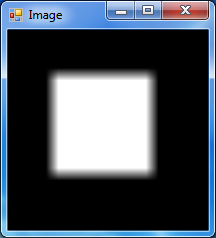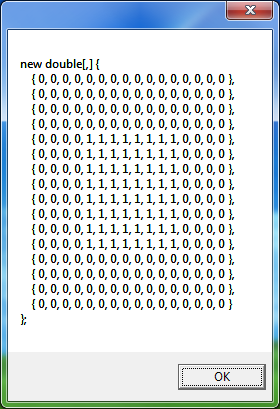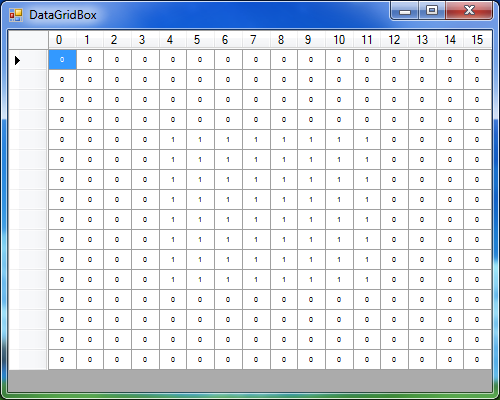﻿ ImageToMatrix Class# ImageToMatrix Class

Bitmap to multidimensional matrix converter.Inheritance Hierarchy
SystemObject
Accord.Imaging.ConvertersImageToMatrix

Namespace:  Accord.Imaging.Converters
Assembly:  Accord.Imaging (in Accord.Imaging.dll) Version: 3.8.0Syntax
```public class ImageToMatrix : IConverter<Bitmap, double[,]>,
IConverter<Bitmap, double[][]>, IConverter<UnmanagedImage, double[,]>,
IConverter<UnmanagedImage, double[][]>, IConverter<Bitmap, float[,]>,
IConverter<Bitmap, float[][]>, IConverter<UnmanagedImage, float[,]>,
IConverter<UnmanagedImage, float[][]>, IConverter<Bitmap, byte[,]>,
IConverter<Bitmap, byte[][]>, IConverter<UnmanagedImage, byte[,]>,
IConverter<UnmanagedImage, byte[][]>, IConverter<Bitmap, double[,,]>,
IConverter<Bitmap, double[][][]>, IConverter<UnmanagedImage, double[,,]>,
IConverter<UnmanagedImage, double[][][]>, IConverter<Bitmap, float[,,]>,
IConverter<Bitmap, float[][][]>, IConverter<UnmanagedImage, float[,,]>,
IConverter<UnmanagedImage, float[][][]>, IConverter<Bitmap, byte[,,]>,
IConverter<Bitmap, byte[][][]>, IConverter<UnmanagedImage, byte[,,]>,
IConverter<UnmanagedImage, byte[][][]>, IConverter<Bitmap, Color[,]>,
IConverter<Bitmap, Color[][]>, IConverter<UnmanagedImage, Color[,]>,
IConverter<UnmanagedImage, Color[][]>```

The ImageToMatrix type exposes the following members.Constructors
NameDescriptionImageToMatrix
Initializes a new instance of the ImageToMatrix class.ImageToMatrix(Double, Double)
Initializes a new instance of the ImageToMatrix class.ImageToMatrix(Double, Double, Int32)
Initializes a new instance of the ImageToMatrix class.
TopProperties
NameDescriptionChannel
Gets or sets the channel to be extracted.Max
Gets or sets the maximum double value in the double array associated with the brightest color.Min
Gets or sets the minimum double value in the double array associated with the darkest color.
TopMethods
NameDescriptionConvert(Bitmap, Byte)
Converts an image from one representation to another.Convert(Bitmap, Byte)
Converts an image from one representation to another.Convert(Bitmap, Byte)
Converts an image from one representation to another.Convert(Bitmap, Byte)
Converts an image from one representation to another.Convert(Bitmap, Double)
Converts an image from one representation to another.Convert(Bitmap, Double)
Converts an image from one representation to another.Convert(Bitmap, Double)
Converts an image from one representation to another.Convert(Bitmap, Double)
Converts an image from one representation to another.Convert(Bitmap, Color)
Converts an image from one representation to another.Convert(Bitmap, Color)
Converts an image from one representation to another.Convert(Bitmap, Single)
Converts an image from one representation to another.Convert(Bitmap, Single)
Converts an image from one representation to another.Convert(Bitmap, Single)
Converts an image from one representation to another.Convert(Bitmap, Single)
Converts an image from one representation to another.Convert(UnmanagedImage, Byte)
Converts an image from one representation to another. When converting to byte, the Max and Min are ignored.Convert(UnmanagedImage, Byte)
Converts an image from one representation to another. When converting to byte, the Max and Min are ignored.Convert(UnmanagedImage, Byte)
Converts an image from one representation to another. When converting to byte, the Max and Min are ignored.Convert(UnmanagedImage, Byte)
Converts an image from one representation to another. When converting to byte, the Max and Min are ignored.Convert(UnmanagedImage, Double)
Converts an image from one representation to another. When converting to byte, the Max and Min are ignored.Convert(UnmanagedImage, Double)
Converts an image from one representation to another.Convert(UnmanagedImage, Double)
Converts an image from one representation to another.Convert(UnmanagedImage, Double)
Converts an image from one representation to another. When converting to byte, the Max and Min are ignored.Convert(UnmanagedImage, Color)
Converts an image from one representation to another. When converting to byte, the Max and Min are ignored.Convert(UnmanagedImage, Color)
Converts an image from one representation to another. When converting to byte, the Max and Min are ignored.Convert(UnmanagedImage, Single)
Converts an image from one representation to another. When converting to byte, the Max and Min are ignored.Convert(UnmanagedImage, Single)
Converts an image from one representation to another.Convert(UnmanagedImage, Single)
Converts an image from one representation to another.Convert(UnmanagedImage, Single)
Converts an image from one representation to another. When converting to byte, the Max and Min are ignored.Equals
Determines whether the specified object is equal to the current object.
(Inherited from Object.)Finalize
Allows an object to try to free resources and perform other cleanup operations before it is reclaimed by garbage collection.
(Inherited from Object.)GetHashCode
Serves as the default hash function.
(Inherited from Object.)GetType
Gets the Type of the current instance.
(Inherited from Object.)MemberwiseClone
Creates a shallow copy of the current Object.
(Inherited from Object.)ToString
Returns a string that represents the current object.
(Inherited from Object.)
TopExtension Methods
NameDescriptionHasMethod
Checks whether an object implements a method with the given name.
(Defined by ExtensionMethods.)IsEqual
Compares two objects for equality, performing an elementwise comparison if the elements are vectors or matrices.
(Defined by Matrix.)To(Type)Overloaded.
Converts an object into another type, irrespective of whether the conversion can be done at compile time or not. This can be used to convert generic types to numeric types during runtime.
(Defined by ExtensionMethods.)ToTOverloaded.
Converts an object into another type, irrespective of whether the conversion can be done at compile time or not. This can be used to convert generic types to numeric types during runtime.
(Defined by ExtensionMethods.)
TopRemarks
This class converts images to multidimensional matrices of either double-precision or single-precision floating-point values.Examples

This example converts a 16x16 Bitmap image into a double[,] array with values between 0 and 1.

```// Obtain an image
// Bitmap image = ...

// Show on screen
ImageBox.Show(image, PictureBoxSizeMode.Zoom);

// Create the converter to convert the image to a
//  matrix containing only values between 0 and 1
ImageToMatrix conv = new ImageToMatrix(min: 0, max: 1);

// Convert the image and store it in the matrix
double[,] matrix; conv.Convert(image, out matrix);

// Show the matrix on screen as an image
ImageBox.Show(matrix, PictureBoxSizeMode.Zoom);```

The resulting image is shown below.Additionally, the image can also be shown in alternative representations such as text or data tables.

```// Show the matrix on screen as a .NET multidimensional array
MessageBox.Show(matrix.ToString(CSharpMatrixFormatProvider.InvariantCulture));

// Show the matrix on screen as a table
DataGridBox.Show(matrix, nonBlocking: true)
.SetAutoSizeColumns(DataGridViewAutoSizeColumnsMode.Fill)See Also